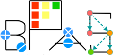Basic Polynomial Algebra Subprograms (BPAS)  v. 1.652
BPASMultivariatePolynomial< Ring, Derived > Member List

This is the complete list of members for BPASMultivariatePolynomial< Ring, Derived >, including all inherited members.

 coefficient(int, const int *) const =0 (defined in BPASMultivariatePolynomial< Ring, Derived >) BPASMultivariatePolynomial< Ring, Derived > pure virtual coefficient(const std::vector< int > &v) const =0 (defined in BPASMultivariatePolynomial< Ring, Derived >) BPASMultivariatePolynomial< Ring, Derived > pure virtual degree(const Symbol &v) const =0 (defined in BPASMultivariatePolynomial< Ring, Derived >) BPASMultivariatePolynomial< Ring, Derived > pure virtual derivative(const Symbol &) const =0 (defined in BPASMultivariatePolynomial< Ring, Derived >) BPASMultivariatePolynomial< Ring, Derived > pure virtual derivative(const Symbol &, int) const =0 (defined in BPASMultivariatePolynomial< Ring, Derived >) BPASMultivariatePolynomial< Ring, Derived > pure virtual differentiate(const Symbol &)=0 (defined in BPASMultivariatePolynomial< Ring, Derived >) BPASMultivariatePolynomial< Ring, Derived > pure virtual differentiate(const Symbol &, int)=0 (defined in BPASMultivariatePolynomial< Ring, Derived >) BPASMultivariatePolynomial< Ring, Derived > pure virtual evaluate(int, const Symbol *, const Ring *) const =0 (defined in BPASMultivariatePolynomial< Ring, Derived >) BPASMultivariatePolynomial< Ring, Derived > pure virtual evaluate(const std::vector< Symbol > &, const std::vector< Ring > &) const =0 (defined in BPASMultivariatePolynomial< Ring, Derived >) BPASMultivariatePolynomial< Ring, Derived > pure virtual numberOfRingVariables() const =0 (defined in BPASMultivariatePolynomial< Ring, Derived >) BPASMultivariatePolynomial< Ring, Derived > pure virtual numberOfVariables() const =0 (defined in BPASMultivariatePolynomial< Ring, Derived >) BPASMultivariatePolynomial< Ring, Derived > pure virtual ringVariables() const =0 (defined in BPASMultivariatePolynomial< Ring, Derived >) BPASMultivariatePolynomial< Ring, Derived > pure virtual setCoefficient(int, const int *, const Ring &r)=0 (defined in BPASMultivariatePolynomial< Ring, Derived >) BPASMultivariatePolynomial< Ring, Derived > pure virtual setCoefficient(const std::vector< int > &v, const Ring &r)=0 (defined in BPASMultivariatePolynomial< Ring, Derived >) BPASMultivariatePolynomial< Ring, Derived > pure virtual setRingVariables(const std::vector< Symbol > &xs)=0 (defined in BPASMultivariatePolynomial< Ring, Derived >) BPASMultivariatePolynomial< Ring, Derived > pure virtual variables() const =0 (defined in BPASMultivariatePolynomial< Ring, Derived >) BPASMultivariatePolynomial< Ring, Derived > pure virtual